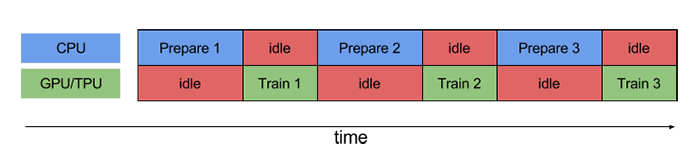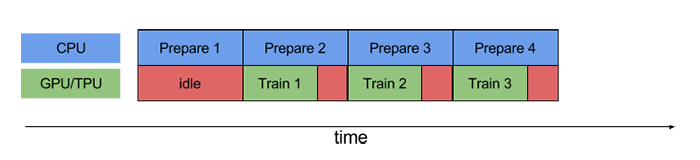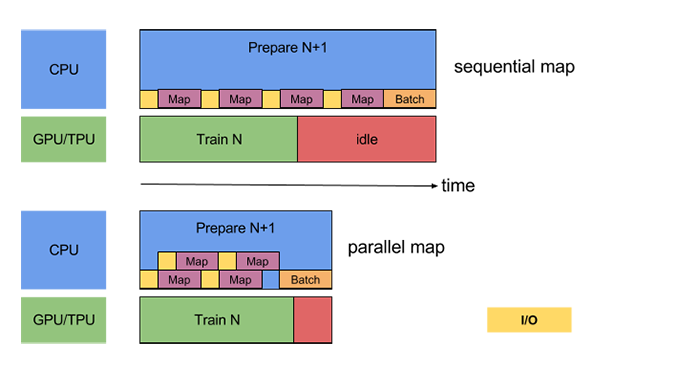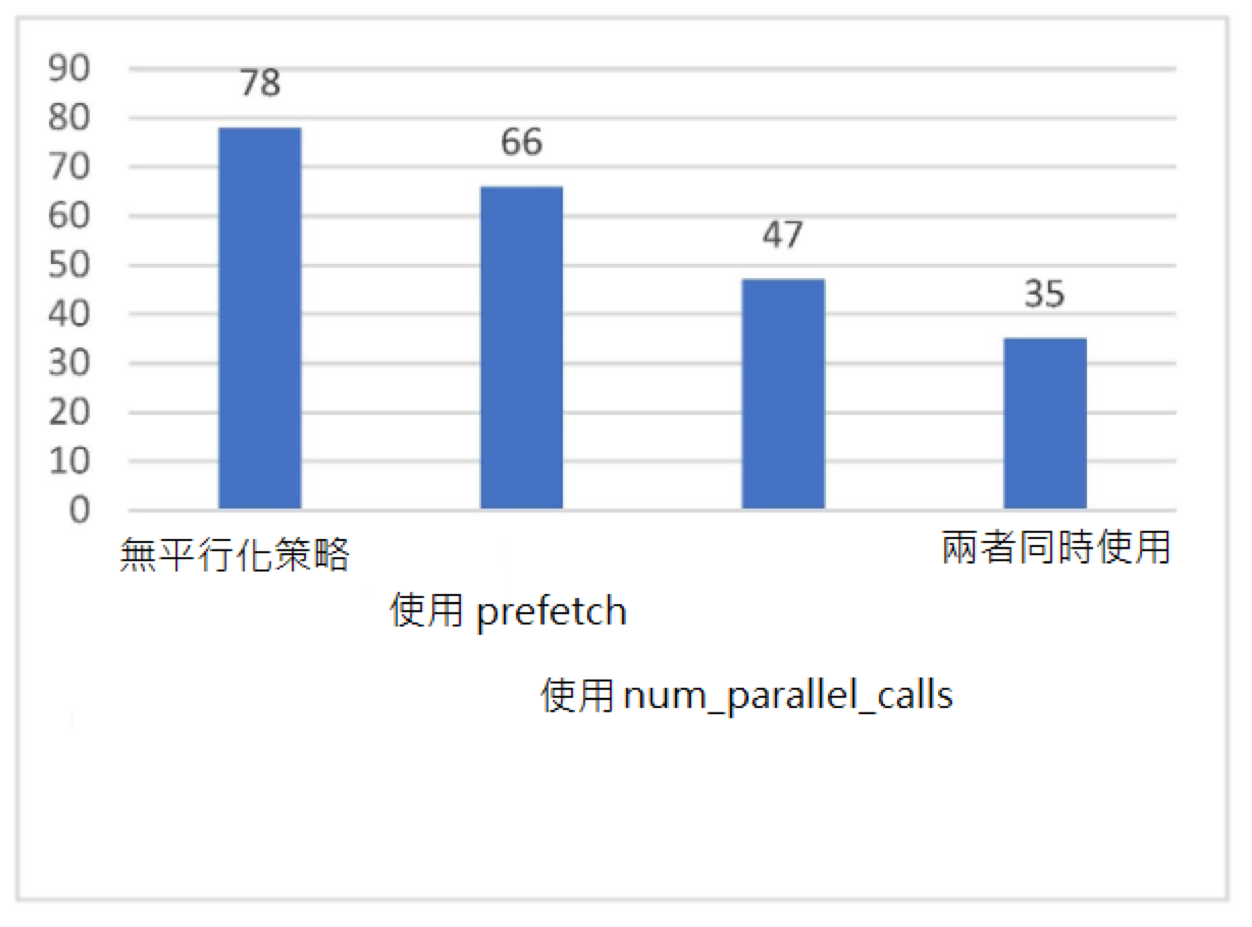# TensorFlow常用模組¶

## `tf.train.Checkpoint` ：變數的保存與還原¶

Checkpoint只保存模型的參數，不保存模型的計算過程，因此一般用於在具有模型程式源碼的時候還原之前訓練好的模型參數。如果需要導出模型（無需程式源程式碼也能運行模型），請參考 “部署”章節中的SavedModel

```checkpoint = tf.train.Checkpoint(model=model)
```

```checkpoint = tf.train.Checkpoint(myAwesomeModel=model, myAwesomeOptimizer=optimizer)
```

```checkpoint.save(save_path_with_prefix)
```

```model_to_be_restored = MyModel()                                        # 待還原參數的同一模型
checkpoint = tf.train.Checkpoint(myAwesomeModel=model_to_be_restored)   # 鍵名保持為“myAwesomeModel”
checkpoint.restore(save_path_with_prefix_and_index)
```

```# train.py 模型訓練階段

model = MyModel()
# 實例化Checkpoint，指定保存對象為model（如果需要保存Optimizer的參數也可加入）
checkpoint = tf.train.Checkpoint(myModel=model)
# ...（模型訓練程式碼）
# 模型訓練完畢後將參數保存到文件（也可以在模型訓練過程中每隔一段時間就保存一次）
checkpoint.save('./save/model.ckpt')
```
```# test.py 模型使用階段

model = MyModel()
checkpoint = tf.train.Checkpoint(myModel=model)             # 實例化Checkpoint，指定還原對象為model
checkpoint.restore(tf.train.latest_checkpoint('./save'))    # 從文件還原模型參數
# 模型使用程式碼
```

`tf.train.Checkpoint` 與以前版本常用的 `tf.train.Saver` 相比，強大之處在於其支援在即時執行模式下“延遲”還原變數。具體而言，當呼叫了 `checkpoint.restore()` ，但模型中的變數還沒有被建立的時候，Checkpoint可以等到變量被建立的時候再進行數值的還原。即時執行模式下，模型中各個層的初始化和變數的建立是在模型第一次被呼叫的時候才進行的（好處在於可以根據輸入的張量形狀而自動確定變量形狀，無需手動指定）。這意味著當模型剛剛被實例化的時候，其實裡面還一個變數都沒有，這時候使用以往的方式去還原變數數值是一定會報錯的。比如，你可以試試在train.py呼叫 `tf.keras.Model``save_weight()` 方法保存model的參數，並在test.py中實例化model後立即調用 `load_weight()` 方法，就會出錯，只有當呼叫了一遍model之後再運行 `load_weight()` 方法才能得到正確的結果。可見， `tf.train.Checkpoint` 在這種情況下可以給我們帶來相當大的便利。另外， `tf.train.Checkpoint` 同時也支持圖執行模式。

```import tensorflow as tf
import numpy as np
import argparse
from zh.model.mnist.mlp import MLP

parser = argparse.ArgumentParser(description='Process some integers.')
args = parser.parse_args()

def train():
model = MLP()
num_batches = int(data_loader.num_train_data // args.batch_size * args.num_epochs)
checkpoint = tf.train.Checkpoint(myAwesomeModel=model)      # 實例化Checkpoint，設置儲存對象為model
for batch_index in range(1, num_batches+1):
y_pred = model(X)
loss = tf.keras.losses.sparse_categorical_crossentropy(y_true=y, y_pred=y_pred)
loss = tf.reduce_mean(loss)
print("batch %d: loss %f" % (batch_index, loss.numpy()))
if batch_index % 100 == 0:                              # 每隔100個Batch儲存一次
path = checkpoint.save('./save/model.ckpt')         # 儲存模型參數到文件
print("model saved to %s" % path)

def test():
model_to_be_restored = MLP()
# 實例化Checkpoint，設置還原對象為新建立的模型model_to_be_restored
checkpoint = tf.train.Checkpoint(myAwesomeModel=model_to_be_restored)
checkpoint.restore(tf.train.latest_checkpoint('./save'))    # 從文件還原模型參數

if __name__ == '__main__':
if args.mode == 'train':
train()
if args.mode == 'test':
test()
```

• 在長時間的訓練後，程式會保存大量的Checkpoint，但我們只想保留最後的幾個Checkpoint；

• Checkpoint預設從1開始編號，每次累加1，但我們可能希望使用別的編號方式（例如使用當前Batch的編號作為文件編號）。

```checkpoint = tf.train.Checkpoint(model=model)
manager = tf.train.CheckpointManager(checkpoint, directory='./save', checkpoint_name='model.ckpt', max_to_keep=k)
```

```import tensorflow as tf
import numpy as np
import argparse
from zh.model.mnist.mlp import MLP

parser = argparse.ArgumentParser(description='Process some integers.')
args = parser.parse_args()

def train():
model = MLP()
num_batches = int(data_loader.num_train_data // args.batch_size * args.num_epochs)
checkpoint = tf.train.Checkpoint(myAwesomeModel=model)
# 使用tf.train.CheckpointManager管理Checkpoint
manager = tf.train.CheckpointManager(checkpoint, directory='./save', max_to_keep=3)
for batch_index in range(1, num_batches):
y_pred = model(X)
loss = tf.keras.losses.sparse_categorical_crossentropy(y_true=y, y_pred=y_pred)
loss = tf.reduce_mean(loss)
print("batch %d: loss %f" % (batch_index, loss.numpy()))
if batch_index % 100 == 0:
# 使用CheckpointManager保存模型參數到文件並自定義編號
path = manager.save(checkpoint_number=batch_index)
print("model saved to %s" % path)

def test():
model_to_be_restored = MLP()
checkpoint = tf.train.Checkpoint(myAwesomeModel=model_to_be_restored)
checkpoint.restore(tf.train.latest_checkpoint('./save'))

if __name__ == '__main__':
if args.mode == 'train':
train()
if args.mode == 'test':
test()
```

## TensorBoard：訓練過程可視化¶

### 實時查看參數變化情況¶

```summary_writer = tf.summary.create_file_writer('./tensorboard')     # 參數為記錄文件所保存的目錄
```

```summary_writer = tf.summary.create_file_writer('./tensorboard')
# 開始模型訓練
for batch_index in range(num_batches):
# ...（訓練程式碼，當前batch的損失值放入變量loss中）
with summary_writer.as_default():                               # 希望使用的記錄器
tf.summary.scalar("loss", loss, step=batch_index)
tf.summary.scalar("MyScalar", my_scalar, step=batch_index)  # 還可以添加其他自定義的變數
```

```tensorboard --logdir=./tensorboard
```

TensorBoard的使用有以下注意事項：

• 如果需要重新訓練，需要刪除掉記錄資料夾內的資訊並重啟TensorBoard（或者建立一個新的記錄資料夾並開啟TensorBoard， `--logdir` 參數設置為新建立的文件夾）；

• 記錄資料夾目錄保持全英文。

### 查看Graph和Profile信息¶

```tf.summary.trace_on(graph=True, profiler=True)  # 開啟Trace，可以記錄圖結構和profile資訊
# 進行訓練
with summary_writer.as_default():
tf.summary.trace_export(name="model_trace", step=0, profiler_outdir=log_dir)    # 保存Trace資訊到文件
```

### 實例：查看多層感知器模型的訓練情況¶

```import tensorflow as tf
from zh.model.mnist.mlp import MLP

num_batches = 1000
batch_size = 50
learning_rate = 0.001
log_dir = 'tensorboard'
model = MLP()
summary_writer = tf.summary.create_file_writer(log_dir)     # 實例化記錄器
tf.summary.trace_on(profiler=True)  # 開啟Trace（可選）
for batch_index in range(num_batches):
y_pred = model(X)
loss = tf.keras.losses.sparse_categorical_crossentropy(y_true=y, y_pred=y_pred)
loss = tf.reduce_mean(loss)
print("batch %d: loss %f" % (batch_index, loss.numpy()))
with summary_writer.as_default():                           # 指定記錄器
tf.summary.scalar("loss", loss, step=batch_index)       # 將目前損失函數的值寫入記錄器
with summary_writer.as_default():
tf.summary.trace_export(name="model_trace", step=0, profiler_outdir=log_dir)    # 儲存Trace資料到文件（可選）
```

## `tf.data` ：資料集的建立與預處理¶

### 資料集對象的建立¶

`tf.data` 的核心是 `tf.data.Dataset` 類別，提供了對資料集的高層封裝。`tf.data.Dataset` 由一系列的可疊代訪問的元素（element）組成，每個元素包含一個或多個張量。比如說，對於一個由圖片組成的資料集，每個元素可以是一個形狀為 `長×寬×通道數` 的圖片張量，也可以是由圖片張量和圖片標籤張量組成的元組（Tuple）。

```import tensorflow as tf
import numpy as np

X = tf.constant([2013, 2014, 2015, 2016, 2017])
Y = tf.constant([12000, 14000, 15000, 16500, 17500])

# 也可以使用NumPy陣列，效果相同
# X = np.array([2013, 2014, 2015, 2016, 2017])
# Y = np.array([12000, 14000, 15000, 16500, 17500])

dataset = tf.data.Dataset.from_tensor_slices((X, Y))

for x, y in dataset:
print(x.numpy(), y.numpy())
```

```2013 12000
2014 14000
2015 15000
2016 16500
2017 17500
```

```import matplotlib.pyplot as plt

(train_data, train_label), (_, _) = tf.keras.datasets.mnist.load_data()
train_data = np.expand_dims(train_data.astype(np.float32) / 255.0, axis=-1)      # [60000, 28, 28, 1]
mnist_dataset = tf.data.Dataset.from_tensor_slices((train_data, train_label))

for image, label in mnist_dataset:
plt.title(label.numpy())
plt.imshow(image.numpy()[:, :, 0])
plt.show()
```

TensorFlow Datasets提供了一個基於 `tf.data.Datasets` 立即使用的資料集集合，相關內容可參考 TensorFlow Datasets 。例如，使用以下語句：

```import tensorflow_datasets as tfds
```

### 資料集對象的預處理¶

`tf.data.Dataset` 類別為我們提供了多種資料集預處理方法。最常使用的如下：

• `Dataset.map(f)` ：對資料集中的每個元素應用函數 `f` ，得到一個新的資料集（這部分往往結合 `tf.io` 進行讀寫和解碼文件， `tf.image` 進行圖片處理）；

• `Dataset.shuffle(buffer_size)` ：將資料集打亂（設定一個固定大小的緩衝區（Buffer），取出前 `buffer_size` 各元素放入，並從緩衝區中隨機採樣，取樣後的資料用後續資料替換）；

• `Dataset.batch(batch_size)` ：將資料集分成批次，即對每 `batch_size` 個元素，使用 `tf.stack()` 在第0維合並，成為一個元素；

```def rot90(image, label):
image = tf.image.rot90(image)
return image, label

mnist_dataset = mnist_dataset.map(rot90)

for image, label in mnist_dataset:
plt.title(label.numpy())
plt.imshow(image.numpy()[:, :, 0])
plt.show()

```

```mnist_dataset = mnist_dataset.batch(4)

for images, labels in mnist_dataset:    # image: [4, 28, 28, 1], labels: 
fig, axs = plt.subplots(1, 4)
for i in range(4):
axs[i].set_title(labels.numpy()[i])
axs[i].imshow(images.numpy()[i, :, :, 0])
plt.show()
```

```mnist_dataset = mnist_dataset.shuffle(buffer_size=10000).batch(4)

for images, labels in mnist_dataset:
fig, axs = plt.subplots(1, 4)
for i in range(4):
axs[i].set_title(labels.numpy()[i])
axs[i].imshow(images.numpy()[i, :, :, 0])
plt.show()
```

`Dataset.shuffle()` 時緩衝區大小 `buffer_size` 的設置

`tf.data.Dataset` 作為一個針對大規模資料設計的疊代器，本身無法方便地獲得自身元素的數量或隨機訪問元素。因此，為了高效且較為充分地打散資料集，需要一些特定的方法。`Dataset.shuffle()` 採取了以下方法：

• 設定一個固定大小為 `buffer_size` 的緩衝區（Buffer）；

• 初始化時，取出資料集中的前 `buffer_size` 個元素放入緩衝區；

• 每次需要從資料集中取元素時，即從緩衝區中隨機採樣一個元素並取出，然後從後續的元素中取出一個放回到之前被取出的位置，以維持緩衝區的大小。

• `buffer_size` 設置為1時，其實等價於沒有進行任何的資料打散；

• 當資料集的標籤順序分佈極為不均勻（例如二元分類時資料集前N個的標籤為0，後N個的標籤為1）時，較小的緩衝區大小會使得訓練時取出的Batch資料很可能全為同一標籤，從而影響訓練效果。一般而言，資料集的順序分佈若較為隨機，則緩衝區的大小可較小，否則則需要設置較大的緩衝區。

### 使用 `tf.data` 的平行化策略提高訓練流程效率¶`Dataset.prefetch()` 的使用方法和前節的 `Dataset.batch()``Dataset.shuffle()` 等非常類似。繼續以前節的MNIST資料集為例，若希望開啟預先加載資料，使用如下程式碼即可：

```mnist_dataset = mnist_dataset.prefetch(buffer_size=tf.data.experimental.AUTOTUNE)
```

```mnist_dataset = mnist_dataset.map(map_func=rot90, num_parallel_calls=2)
```### 資料集元素的獲取與使用¶

```dataset = tf.data.Dataset.from_tensor_slices((A, B, C, ...))
for a, b, c, ... in dataset:
# 對張量a, b, c等進行操作，例如送入模型進行訓練
```

```dataset = tf.data.Dataset.from_tensor_slices((A, B, C, ...))
it = iter(dataset)
a_0, b_0, c_0, ... = next(it)
a_1, b_1, c_1, ... = next(it)
```

Keras支援使用 `tf.data.Dataset` 直接作為輸入。當呼叫 `tf.keras.Model``fit()``evaluate()` 方法時，可以將參數中的輸入資料 `x` 指定為一個元素格式為 `(輸入資料, 標籤資料)``Dataset` ，並忽略掉參數中的標籤資料 `y` 。例如，對於上述的MNIST資料集，常規的Keras訓練方式是：

```model.fit(x=train_data, y=train_label, epochs=num_epochs, batch_size=batch_size)
```

```model.fit(mnist_dataset, epochs=num_epochs)
```

### 實例：cats_vs_dogs圖片分類¶

```import tensorflow as tf
import os

num_epochs = 10
batch_size = 32
learning_rate = 0.001
data_dir = 'C:/datasets/cats_vs_dogs'
train_cats_dir = data_dir + '/train/cats/'
train_dogs_dir = data_dir + '/train/dogs/'
test_cats_dir = data_dir + '/valid/cats/'
test_dogs_dir = data_dir + '/valid/dogs/'

def _decode_and_resize(filename, label):
image_decoded = tf.image.decode_jpeg(image_string)  # 解碼JPEG圖片
image_resized = tf.image.resize(image_decoded, [256, 256]) / 255.0
return image_resized, label

if __name__ == '__main__':
# 建構訓練資料集
train_cat_filenames = tf.constant([train_cats_dir + filename for filename in os.listdir(train_cats_dir)])
train_dog_filenames = tf.constant([train_dogs_dir + filename for filename in os.listdir(train_dogs_dir)])
train_filenames = tf.concat([train_cat_filenames, train_dog_filenames], axis=-1)
train_labels = tf.concat([
tf.zeros(train_cat_filenames.shape, dtype=tf.int32),
tf.ones(train_dog_filenames.shape, dtype=tf.int32)],
axis=-1)

train_dataset = tf.data.Dataset.from_tensor_slices((train_filenames, train_labels))
train_dataset = train_dataset.map(
map_func=_decode_and_resize,
num_parallel_calls=tf.data.experimental.AUTOTUNE)
# 取出前buffer_size個資料放入buffer，並從其中隨機取樣，取樣後的資料用後續資料替換
train_dataset = train_dataset.shuffle(buffer_size=23000)
train_dataset = train_dataset.batch(batch_size)
train_dataset = train_dataset.prefetch(tf.data.experimental.AUTOTUNE)

model = tf.keras.Sequential([
tf.keras.layers.Conv2D(32, 3, activation='relu', input_shape=(256, 256, 3)),
tf.keras.layers.MaxPooling2D(),
tf.keras.layers.Conv2D(32, 5, activation='relu'),
tf.keras.layers.MaxPooling2D(),
tf.keras.layers.Flatten(),
tf.keras.layers.Dense(64, activation='relu'),
tf.keras.layers.Dense(2, activation='softmax')
])

model.compile(
loss=tf.keras.losses.sparse_categorical_crossentropy,
metrics=[tf.keras.metrics.sparse_categorical_accuracy]
)

model.fit(train_dataset, epochs=num_epochs)
```

```    # 建構測試資料集
test_cat_filenames = tf.constant([test_cats_dir + filename for filename in os.listdir(test_cats_dir)])
test_dog_filenames = tf.constant([test_dogs_dir + filename for filename in os.listdir(test_dogs_dir)])
test_filenames = tf.concat([test_cat_filenames, test_dog_filenames], axis=-1)
test_labels = tf.concat([
tf.zeros(test_cat_filenames.shape, dtype=tf.int32),
tf.ones(test_dog_filenames.shape, dtype=tf.int32)],
axis=-1)

test_dataset = tf.data.Dataset.from_tensor_slices((test_filenames, test_labels))
test_dataset = test_dataset.map(_decode_and_resize)
test_dataset = test_dataset.batch(batch_size)

print(model.metrics_names)
print(model.evaluate(test_dataset))
```tf.data 的平行化策略性能測試（縱軸為每epoch訓練所需時間，單位：秒）

## TFRecord ：TensorFlow資料集存儲格式¶

TFRecord 是TensorFlow 中的資料集存儲格式。當我們將資料集整理成 TFRecord 格式後，TensorFlow就可以高效地讀取和處理這些資料集，從而幫助我們更高效地進行大規模的模型訓練。

TFRecord可以理解為一系列序列化的 `tf.train.Example` 元素所組成的列表文件，而每一個 `tf.train.Example` 又由若干個 `tf.train.Feature` 的字典組成。形式如下：

```# dataset.tfrecords
[
{   # example 1 (tf.train.Example)
'feature_1': tf.train.Feature,
...
'feature_k': tf.train.Feature
},
...
{   # example N (tf.train.Example)
'feature_1': tf.train.Feature,
...
'feature_k': tf.train.Feature
}
]
```

• 讀取該資料元素到內存；

• 將該元素轉換為 `tf.train.Example` 對象（每一個 `tf.train.Example` 由若干個 `tf.train.Feature` 的字典組成，因此需要先建立Feature的字典）；

• 將該 `tf.train.Example` 對象序列化為字串，並通過一個預先定義的 `tf.io.TFRecordWriter` 寫入 TFRecord 文件。

• 通過 `tf.data.TFRecordDataset` 讀入原始的 TFRecord 文件（此時文件中的 `tf.train.Example` 對象尚未被反序列化），獲得一個 `tf.data.Dataset` 資料集對象；

• 通過 `Dataset.map` 方法，對該資料集對象中的每一個序列化的 `tf.train.Example` 字串執行 `tf.io.parse_single_example` 函數，從而實現反序列化。

### 將資料集存儲為 TFRecord 文件¶

```import tensorflow as tf
import os

data_dir = 'C:/datasets/cats_vs_dogs'
train_cats_dir = data_dir + '/train/cats/'
train_dogs_dir = data_dir + '/train/dogs/'
tfrecord_file = data_dir + '/train/train.tfrecords'

train_cat_filenames = [train_cats_dir + filename for filename in os.listdir(train_cats_dir)]
train_dog_filenames = [train_dogs_dir + filename for filename in os.listdir(train_dogs_dir)]
train_filenames = train_cat_filenames + train_dog_filenames
train_labels =  * len(train_cat_filenames) +  * len(train_dog_filenames)  # 將 cat 類的標籤設為0，dog 類的標籤設為1
```

```with tf.io.TFRecordWriter(tfrecord_file) as writer:
for filename, label in zip(train_filenames, train_labels):
image = open(filename, 'rb').read()     # 讀取資料集圖片到內存，image 為一個
feature = {                             # 建立 tf.train.Feature 字典
'image': tf.train.Feature(bytes_list=tf.train.BytesList(value=[image])),  # 圖片是一個 Bytes 對象
'label': tf.train.Feature(int64_list=tf.train.Int64List(value=[label]))   # 標籤是一個 Int 對象
}
example = tf.train.Example(features=tf.train.Features(feature=feature)) # 通過字典建立 Example
writer.write(example.SerializeToString())   # 將Example序列化並寫入 TFRecord 文件
```

• `tf.train.BytesList` ：字元串或原始Byte文件（如圖片），通過 `bytes_list` 參數傳入一個由字串數組初始化的 `tf.train.BytesList` 對象；

• `tf.train.FloatList` ：浮點數，通過 `float_list` 參數傳入一個由浮點數陣列初始化的 `tf.train.FloatList` 對象；

• `tf.train.Int64List` ：整數，通過 `int64_list` 參數傳入一個由整數陣列初始化的 `tf.train.Int64List` 對象。

### 讀取 TFRecord 文件¶

```raw_dataset = tf.data.TFRecordDataset(tfrecord_file)    # 讀取 TFRecord 文件

feature_description = { # 定義Feature結構，告訴解碼器每個Feature的類型是什麼
'image': tf.io.FixedLenFeature([], tf.string),
'label': tf.io.FixedLenFeature([], tf.int64),
}

def _parse_example(example_string): # 將 TFRecord 文件中的每一個序列化的 tf.train.Example 解碼
feature_dict = tf.io.parse_single_example(example_string, feature_description)
feature_dict['image'] = tf.io.decode_jpeg(feature_dict['image'])    # 解碼JPEG圖片
return feature_dict['image'], feature_dict['label']

dataset = raw_dataset.map(_parse_example)
```

```import matplotlib.pyplot as plt

for image, label in dataset:
plt.title('cat' if label == 0 else 'dog')
plt.imshow(image.numpy())
plt.show()
```

## `tf.function` ：圖執行模式 *¶

### `tf.function` 基礎使用方法¶

```import tensorflow as tf
import time
from zh.model.mnist.cnn import CNN

num_batches = 1000
batch_size = 50
learning_rate = 0.001
model = CNN()

@tf.function
def train_one_step(X, y):
y_pred = model(X)
loss = tf.keras.losses.sparse_categorical_crossentropy(y_true=y, y_pred=y_pred)
loss = tf.reduce_mean(loss)
# 注意這裡使用了TensorFlow內建的tf.print()。@tf.function不支援Python內建的print方法
tf.print("loss", loss)

start_time = time.time()
for batch_index in range(num_batches):
train_one_step(X, y)
end_time = time.time()
print(end_time - start_time)
```

### `tf.function` 內在機制¶

• 在即時執行模式關閉的環境下，函數內的程式碼依次運行。也就是說，每個 `tf.` 方法都只是定義了計算節點，而並沒有進行任何實質的計算。這與TensorFlow 1.X的圖執行模式是一致的；

• 使用AutoGraph將函數中的Python控制語句轉換成TensorFlow計算圖中的對應節點（比如說 `while``for` 語句轉換為 `tf.while``if` 語句轉換為 `tf.cond` 等等；

• 基於上面的兩步，建立函數內程式碼的計算圖表示（為了保證圖的計算順序，圖中還會自動加入一些 `tf.control_dependencies` 節點）；

• 運行一次這個計算圖；

• 基於函數的名字和輸入的函數參數的類型生成一個雜湊值，並將建立的計算圖緩衝區到一個雜湊表中。

```graph = train_one_step.get_concrete_function(X, y)
```

```import tensorflow as tf
import numpy as np

@tf.function
def f(x):
print("The function is running in Python")
tf.print(x)

a = tf.constant(1, dtype=tf.int32)
f(a)
b = tf.constant(2, dtype=tf.int32)
f(b)
b_ = np.array(2, dtype=np.int32)
f(b_)
c = tf.constant(0.1, dtype=tf.float32)
f(c)
d = tf.constant(0.2, dtype=tf.float32)
f(d)
```

```The function is running in Python
1
2
2
The function is running in Python
0.1
0.2
```

• 將函數內的程式碼依次運行了一遍（因此輸出了文件）；

• 構建了計算圖，然後運行了一次該計算圖（因此輸出了1）。這里 `tf.print(x)` 可以作為計算圖的節點，但Python內建的 `print` 則不能被轉換成計算圖的節點。因此，計算圖中只包含了 `tf.print(x)` 這一操作；

• 將該計算圖緩衝區到了一個雜湊表中（如果之後再有類型為 `tf.int32` ，shape為空的張量輸入，則重復使用已建構的計算圖）。

```f(d)
f(1)
f(2)
f(1)
f(0.1)
f(0.2)
f(0.1)
```

```The function is running in Python
1
The function is running in Python
2
1
The function is running in Python
0.1
The function is running in Python
0.2
0.1
```

```import tensorflow as tf

a = tf.Variable(0.0)

@tf.function
def g():
a.assign(a + 1.0)
return a

print(g())
print(g())
print(g())
```

```tf.Tensor(1.0, shape=(), dtype=float32)
tf.Tensor(2.0, shape=(), dtype=float32)
tf.Tensor(3.0, shape=(), dtype=float32)
```

### AutoGraph：將Python控制流轉換為TensorFlow計算圖¶

```import tensorflow as tf

@tf.function
def square_if_positive(x):
if x > 0:
x = x * x
else:
x = 0
return x

a = tf.constant(1)
b = tf.constant(-1)
print(square_if_positive(a), square_if_positive(b))
print(tf.autograph.to_code(square_if_positive.python_function))
```

```tf.Tensor(1, shape=(), dtype=int32) tf.Tensor(0, shape=(), dtype=int32)
def tf__square_if_positive(x):
do_return = False
retval_ = ag__.UndefinedReturnValue()
cond = x > 0

def get_state():
return ()

def set_state(_):
pass

def if_true():
x_1, = x,
x_1 = x_1 * x_1
return x_1

def if_false():
x = 0
return x
x = ag__.if_stmt(cond, if_true, if_false, get_state, set_state)
do_return = True
retval_ = x
cond_1 = ag__.is_undefined_return(retval_)

def get_state_1():
return ()

def set_state_1(_):
pass

def if_true_1():
retval_ = None
return retval_

def if_false_1():
return retval_
retval_ = ag__.if_stmt(cond_1, if_true_1, if_false_1, get_state_1, set_state_1)
return retval_
```

### 使用傳統的 `tf.Session`¶

```    optimizer = tf.compat.v1.train.AdamOptimizer(learning_rate=learning_rate)
num_batches = int(data_loader.num_train_data // batch_size * num_epochs)
# 建立計算圖
X_placeholder = tf.compat.v1.placeholder(name='X', shape=[None, 28, 28, 1], dtype=tf.float32)
y_placeholder = tf.compat.v1.placeholder(name='y', shape=[None], dtype=tf.int32)
y_pred = model(X_placeholder)
loss = tf.keras.losses.sparse_categorical_crossentropy(y_true=y_placeholder, y_pred=y_pred)
loss = tf.reduce_mean(loss)
train_op = optimizer.minimize(loss)
sparse_categorical_accuracy = tf.keras.metrics.SparseCategoricalAccuracy()
# 建立Session
with tf.compat.v1.Session() as sess:
sess.run(tf.compat.v1.global_variables_initializer())
for batch_index in range(num_batches):
# 使用Session.run()將資料送入計算圖節點，進行訓練以及計算損失函數
_, loss_value = sess.run([train_op, loss], feed_dict={X_placeholder: X, y_placeholder: y})
print("batch %d: loss %f" % (batch_index, loss_value))

for batch_index in range(num_batches):
start_index, end_index = batch_index * batch_size, (batch_index + 1) * batch_size
print("test accuracy: %f" % sess.run(sparse_categorical_accuracy.result()))
```

## `tf.TensorArray` ：TensorFlow 動態陣列 *¶

• `arr = tf.TensorArray(dtype, size, dynamic_size=False)` ：宣告一個大小為 `size` ，類型為 `dtype` 的TensorArray `arr` 。如果將 `dynamic_size` 參數設置為 `True` ，則該陣列會自動增長空間。

• `write(index, value)` ：將 `value` 寫入陣列的第 `index` 個位置；

• `read(index)` ：讀取陣列的第 `index` 個值；

```arr = arr.write(index, value)
```

```arr.write(index, value)     # 生成的計算圖操作沒有變數接收，從而丟失
```

```import tensorflow as tf

@tf.function
arr = tf.TensorArray(dtype=tf.float32, size=3)
arr = arr.write(0, tf.constant(0.0))
arr = arr.write(1, tf.constant(1.0))
arr = arr.write(2, tf.constant(2.0))
return arr_0, arr_1, arr_2

print(a, b, c)
```

```tf.Tensor(0.0, shape=(), dtype=float32) tf.Tensor(1.0, shape=(), dtype=float32) tf.Tensor(2.0, shape=(), dtype=float32)
```

## `tf.config`：GPU的使用與分配 *¶

### 指定當前程式使用的GPU¶

```gpus = tf.config.list_physical_devices(device_type='GPU')
cpus = tf.config.list_physical_devices(device_type='CPU')
print(gpus, cpus)
```

```[PhysicalDevice(name='/physical_device:GPU:0', device_type='GPU'),
PhysicalDevice(name='/physical_device:GPU:1', device_type='GPU'),
PhysicalDevice(name='/physical_device:GPU:2', device_type='GPU'),
PhysicalDevice(name='/physical_device:GPU:3', device_type='GPU')]
[PhysicalDevice(name='/physical_device:CPU:0', device_type='CPU')]
```

```gpus = tf.config.list_physical_devices(device_type='GPU')
tf.config.set_visible_devices(devices=gpus[0:2], device_type='GPU')
```

```export CUDA_VISIBLE_DEVICES=2,3
```

```import os
os.environ['CUDA_VISIBLE_DEVICES'] = "2,3"
```

### 設置顯示卡記憶體儲存空間使用策略¶

• 僅在需要時申請顯示卡記憶體儲存空間（程式初始運行時消耗很少的顯示卡儲存空間，隨著程式的運行而動態申請顯示卡記憶體儲存空間）；

• 限制消耗固定大小的顯示卡記憶體儲存空間（程式不會超出限定的顯示卡記憶體儲存大小，若超出的報錯）。

```gpus = tf.config.list_physical_devices(device_type='GPU')
for gpu in gpus:
tf.config.experimental.set_memory_growth(device=gpu, enable=True)
```

```gpus = tf.config.list_physical_devices(device_type='GPU')
tf.config.set_logical_device_configuration(
gpus,
[tf.config.LogicalDeviceConfiguration(memory_limit=1024)])
```

TensorFlow 1.X 的 圖執行模式 下，可以在實例化新的session時傳入 `tf.compat.v1.ConfigPhoto` 類別來設置TensorFlow使用顯示卡記憶體儲存的策略。具體方式是實例化一個 `tf.ConfigProto` 類別，設置參數，並在創建 `tf.compat.v1.Session` 時指定Config參數。以下程式碼通過 `allow_growth` 選項設置TensorFlow僅在需要時申請顯示卡記憶體儲存空間：

```config = tf.compat.v1.ConfigProto()
config.gpu_options.allow_growth = True
sess = tf.compat.v1.Session(config=config)
```

```config = tf.compat.v1.ConfigProto()
config.gpu_options.per_process_gpu_memory_fraction = 0.4
tf.compat.v1.Session(config=config)
```

### 單GPU模擬多GPU環境¶

```gpus = tf.config.list_physical_devices('GPU')
tf.config.set_logical_device_configuration(
gpus,
[tf.config.LogicalDeviceConfiguration(memory_limit=2048),
tf.config.LogicalDeviceConfiguration(memory_limit=2048)])
```

```Number of devices: 2
```